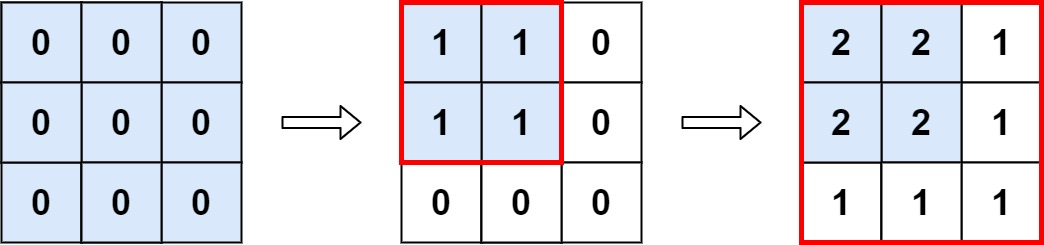### LeetCode• ㊗️
• 大家
• offer
• 多多！

## Problem

You are given an m x n matrix M initialized with all 0’s and an array of operations ops, where ops[i] = [ai, bi] means M[x][y] should be incremented by one for all 0 <= x < ai and 0 <= y < bi.

Count and return the number of maximum integers in the matrix after performing all the operations.

Example 1:Input: m = 3, n = 3, ops = [[2,2],[3,3]]
Output: 4
Explanation: The maximum integer in M is 2, and there are four of it in M. So return 4.


Example 2:

Input: m = 3, n = 3, ops = [[2,2],[3,3],[3,3],[3,3],[2,2],[3,3],[3,3],[3,3],[2,2],[3,3],[3,3],[3,3]]
Output: 4


Example 3:

Input: m = 3, n = 3, ops = []
Output: 9


Constraints:

• $1 <= m, n <= 4 * 10^4$
• $0 <= ops.length <= 10^4$
• ops[i].length == 2
• $1 <= a_i <= m$
• $1 <= b_i <= n$

## Code

class Solution {
public int maxCount(int m, int n, int[][] ops) {
int minx = m;
int miny = n;

for(int[] op : ops) {
minx = Math.min(minx, op);
miny = Math.min(miny, op);
}

return minx * miny;
}
}